# Printable Bubble Numbers 1-10Updated on Jun 08, 2023By Printablee Team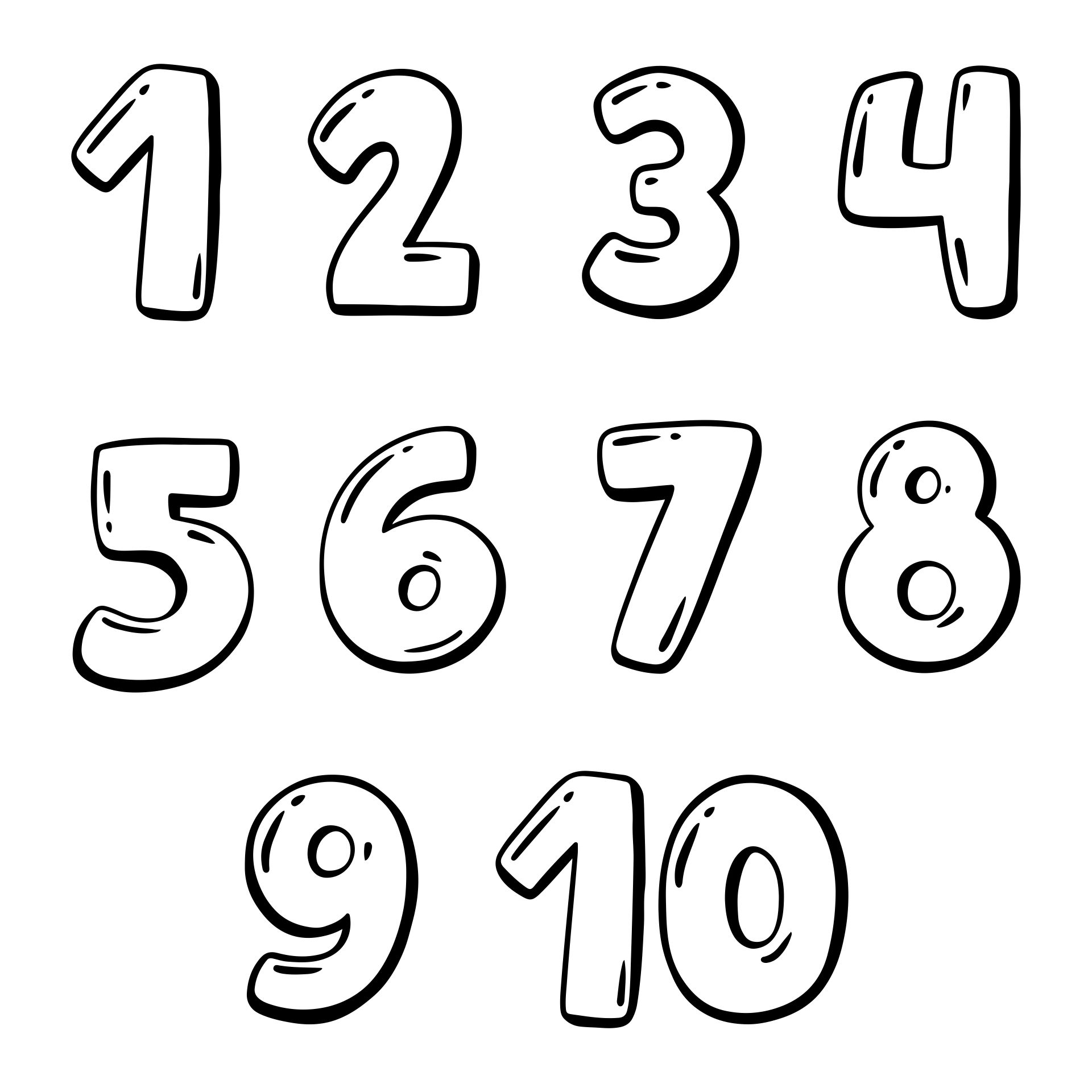## How is Number Sense Related to Spatial Thinking Skills?

A person's capacity to comprehend and interact with numbers in a flexible, intuitive, and meaningful way is referred to as having "number sense," which is a fundamental mathematical concept. It is a crucial ability that lays the groundwork for mathematical success because it enables students to comprehend the relationships between numbers, spot patterns, and connections, and use this understanding to address problems in the real world.

Numerous mathematical skills, such as estimation, comparison, ordering, and manipulation of numbers are all part of number sense. Understanding mathematical ideas like place value, fractions, decimals, and percentages is also necessary.

Fundamentally, having a strong grasp of the dimensions and connections between numbers is known as having number sense. Basic knowledge of addition, subtraction, multiplication, and division is required as well as an awareness of how these operations relate to one another.

Because it requires a grasp of the spatial connections between numbers and their representations, number sense is intimately related to spatial thinking skills. This involves comprehending number lines, arrays, graphs, and other numerical visual representations.

### What are the misconceptions about number sense?

Learning arithmetic facts is only one aspect of developing number awareness. While memorizing arithmetic facts is crucial, developing number sense requires much more than that. It requires comprehending how numbers relate to one another and being able to apply that understanding to issues.

Not just students who are skilled at arithmetic should have number sense. With practice and instruction, one can gradually hone their sense of numbers. Every level of math needs number sense. Number sense is crucial for math students at all levels since it aids in the understanding of numbers and the development of problem-solving abilities.

It takes more than simply having a fast memory for math to have number sense. Although mental computation is a crucial element of number sense, it is not the sole one. Aside from estimation skills and problem-solving techniques, number sense also includes an understanding of how numbers relate to one another.

A characteristic that may be developed is number sense. With practice and instruction, one may gradually hone their sense of numbers.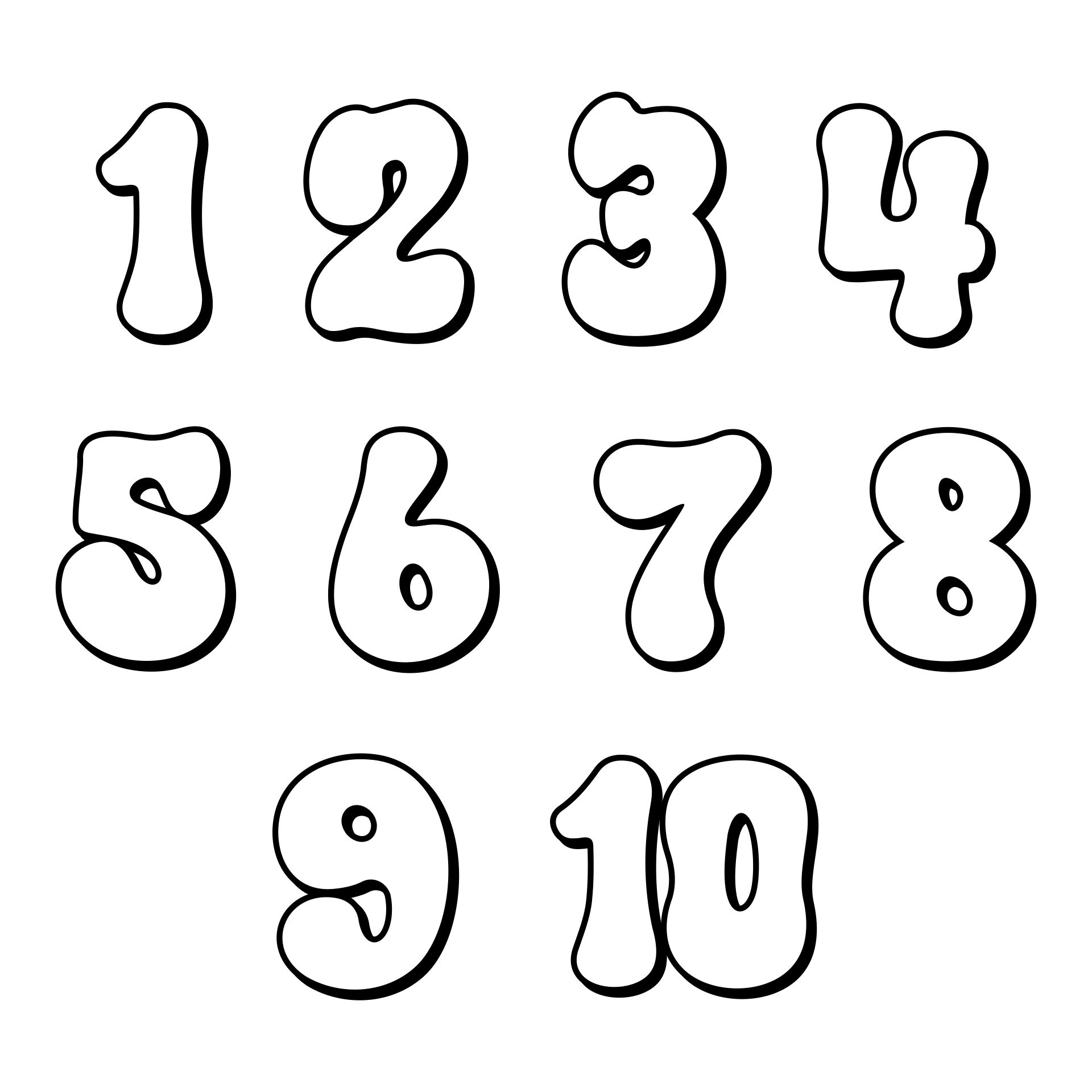We also have more printable number you may like:
Printable Number Line 1 10
2 Inch Printable Numbers
Printable Numbers 1- 30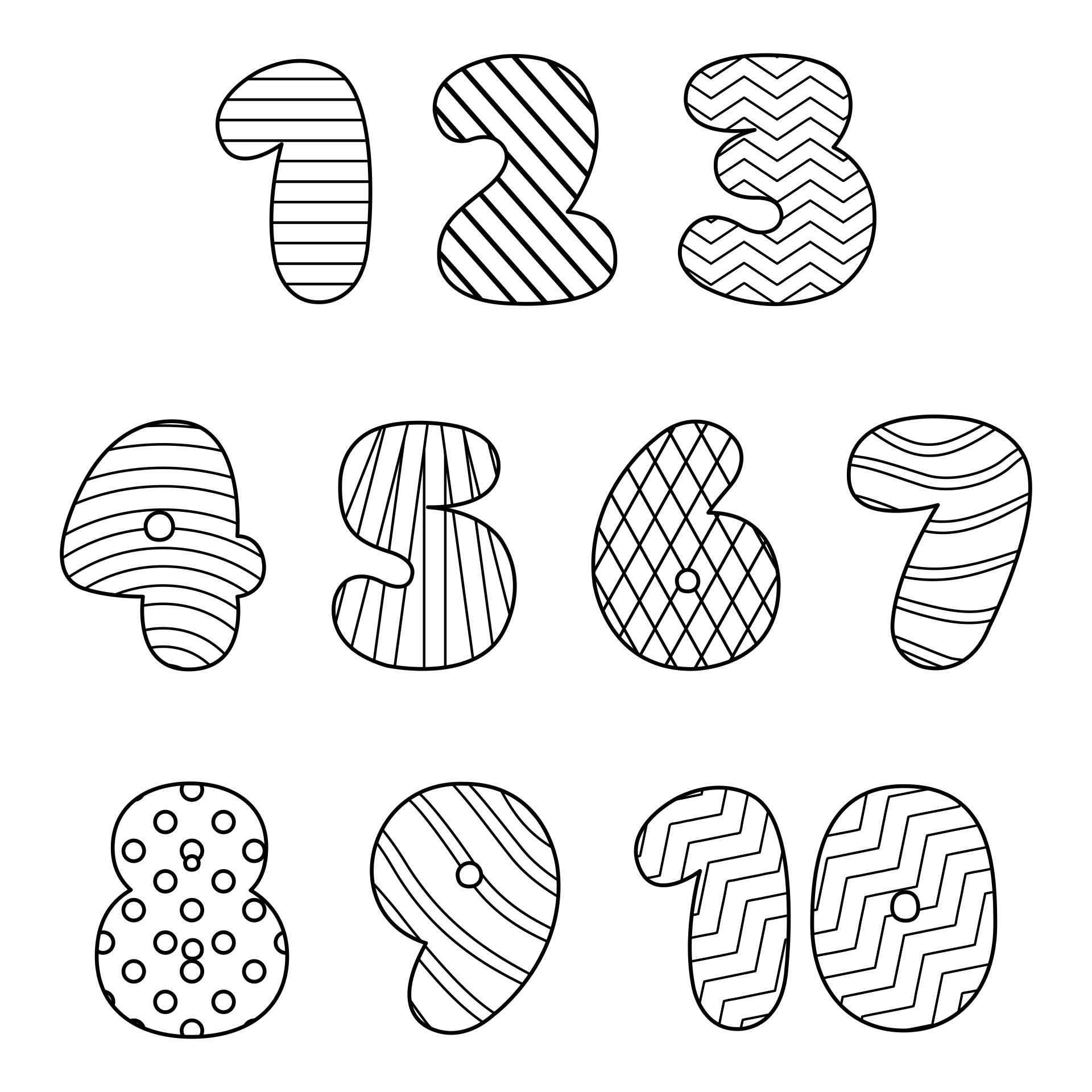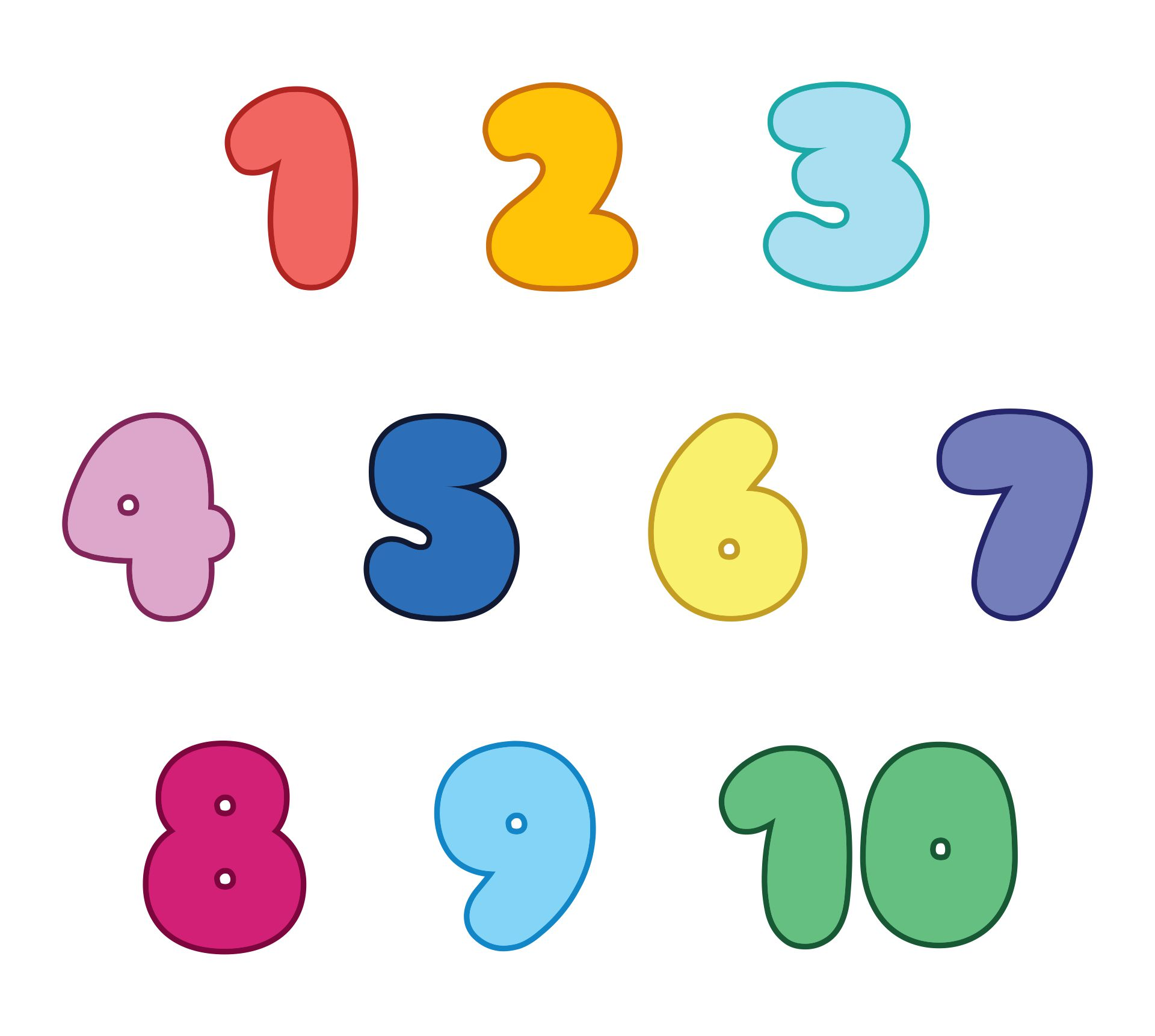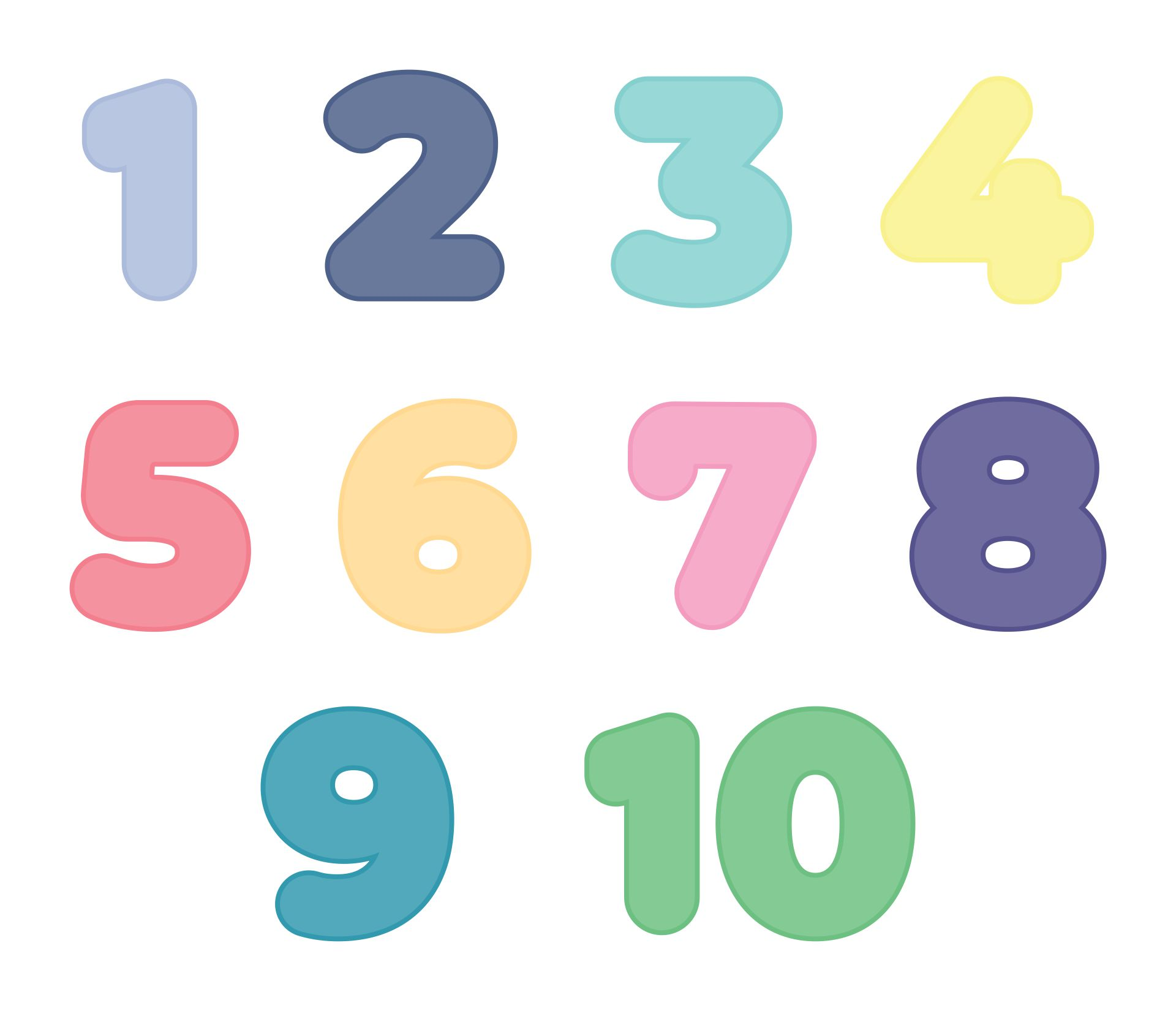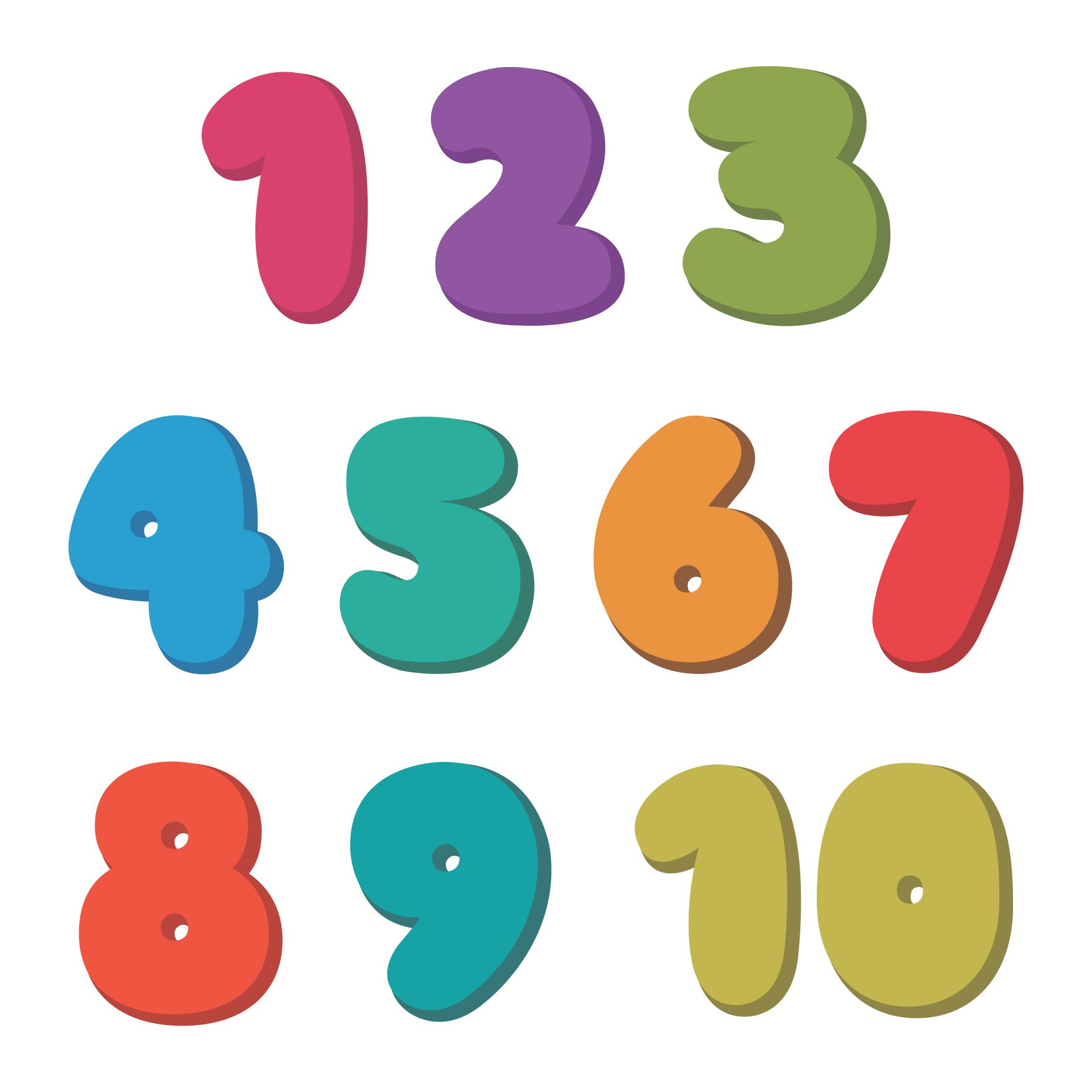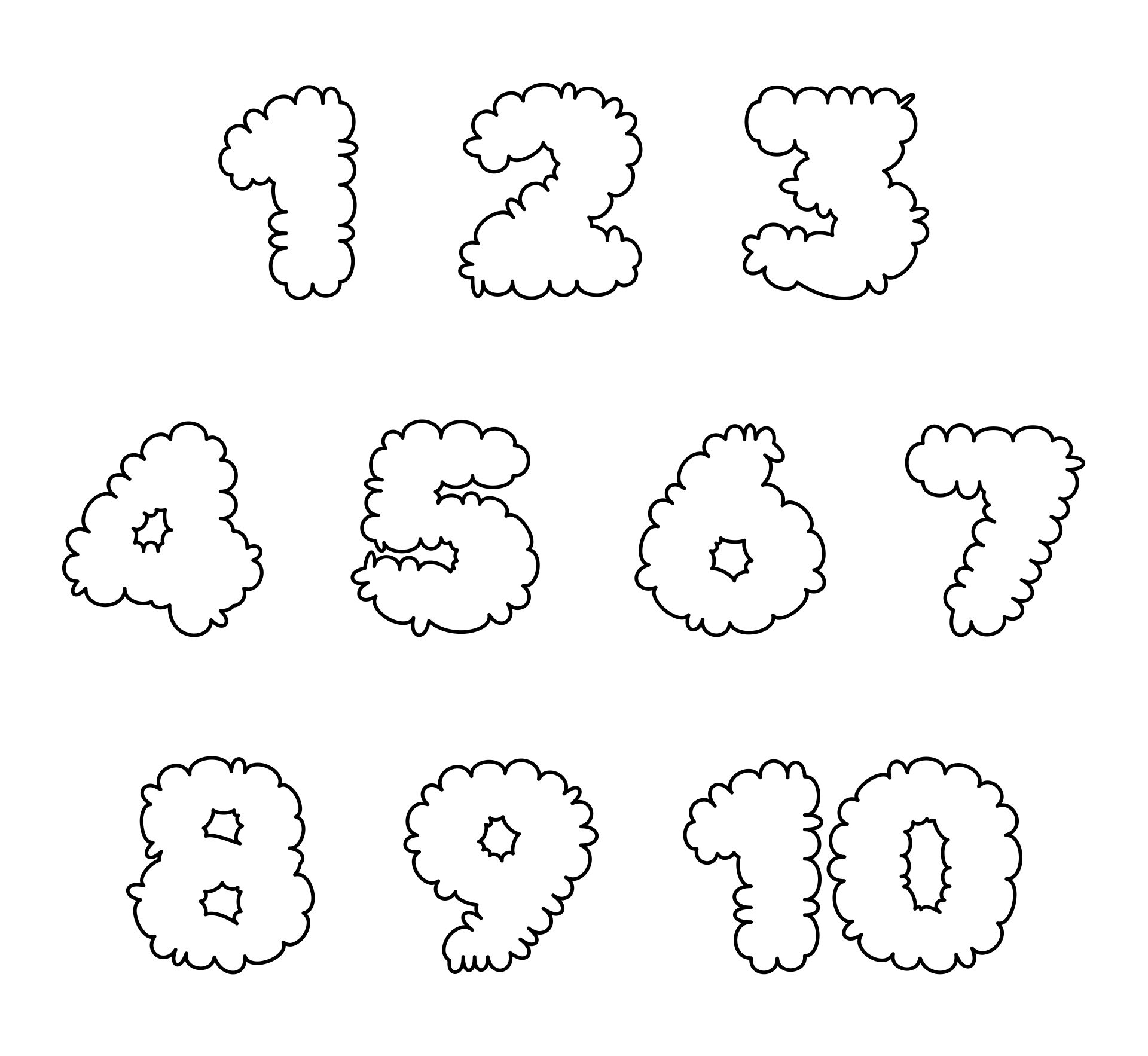## Why do Math Lessons need to be Incorporated with Games?

Among other things, effective math games should follow the math curriculum and learning goals. Games should be developed to focus on specific arithmetic ideas or skills that students are expected to master, and they should be utilized as a supplement rather than a replacement for traditional training. This ensures that the games are instructive and are successfully included in the overall arithmetic program.

Math games must be developed to challenge students and promote critical thinking. Games that require little thought or are overly basic will not promote good learning. Games should be developed to challenge children's critical thinking, encourage creative math applications, and strengthen their problem-solving skills.

It's important to consider how interactive math games can be. Effective math games should be very interactive so that students can engage with the material and learn by doing. In order for players to grow in comprehension of mathematical ideas and learn from their mistakes, games should include opportunities for reflection and feedback.

Successful math games should promote collaboration and social learning. Through teamwork, idea sharing, and mutual learning, students may participate in group activities and games. This might promote deeper learning and create a joyful learning environment.

## What are some activities that can help develop number sense?

Exploration and problem-solving activities can help pupils improve their numerical sense. Students may get a better grasp of number connections and acquire the skills needed to excel in math and beyond by engaging with numbers in a variety of ways.

### 1. Number Talks

In this exercise, students collaborate to solve arithmetic problems while discussing various mental math techniques. It aids in the growth of pupils' mental agility and capacity for original numerical thought.

### 2. Estimation Activities

Activities involving estimation include calculating the volume of a container or the length of an object. By enabling pupils to distinguish between what is reasonable and what is not, estimation aids in kids' development of number sense.

### 3. Math Games

In a fun and interesting fashion, games like "Math War" or "Math Dice" can help pupils exercise their mental math abilities and improve their number sense.

### 4. Number Line Activities

By using number lines, students can practice counting, addition, and subtraction as well as understand the relationships between different numbers.

### 5. Activities With Word Problems

Word problems can assist students in refining their problem-solving techniques and applying their number sense to practical circumstances.

### 6. Activities with Base Ten Blocks

Using base ten blocks can assist kids comprehend place value and numerical relationships.

### 7. Fraction Activities

Fraction-matching games and fraction pizza can assist children grasp fractions and their links to whole numbers.